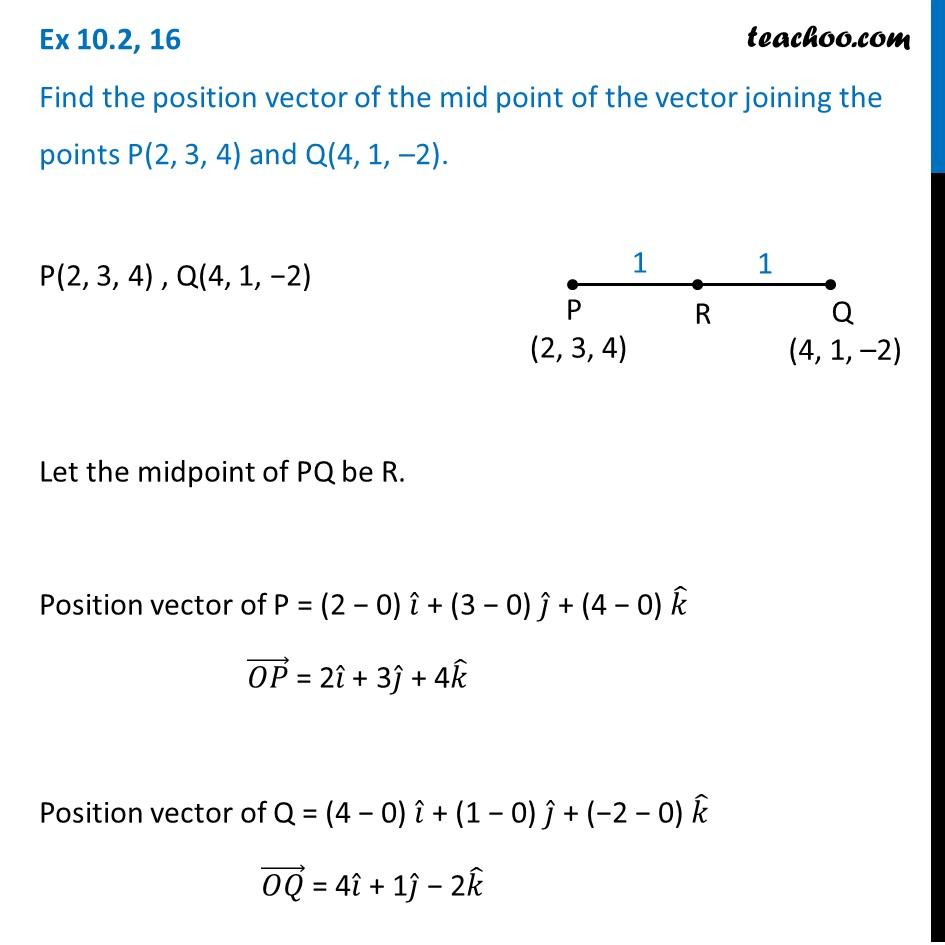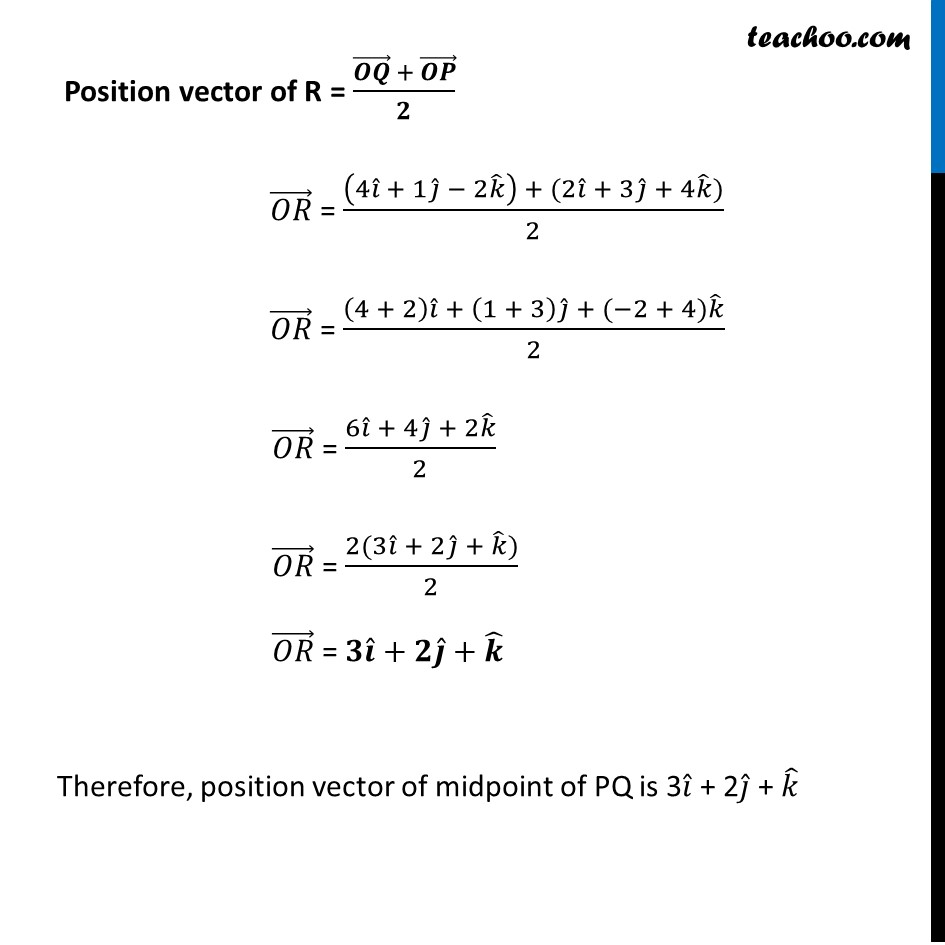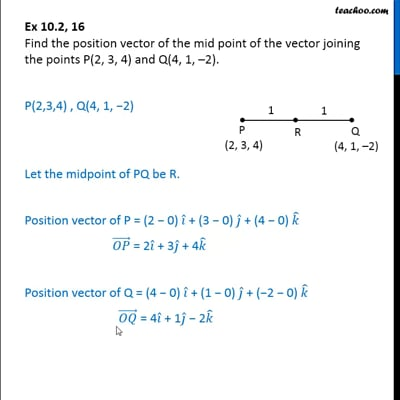Ex 10.2

Chapter 10 Class 12 Vector Algebra
Serial order wiseThis video is only available for Teachoo black users

Solve all your doubts with Teachoo Black (new monthly pack available now!)

### Transcript

Ex 10.2, 16 Find the position vector of the mid point of the vector joining the points P(2, 3, 4) and Q(4, 1, –2). P(2, 3, 4) , Q(4, 1, −2) Let the midpoint of PQ be R. Position vector of P = (2 − 0) 𝑖 ̂ + (3 − 0) 𝑗 ̂ + (4 − 0) 𝑘 ̂ (𝑂𝑃) ⃗ = 2𝑖 ̂ + 3𝑗 ̂ + 4𝑘 ̂ Position vector of Q = (4 − 0) 𝑖 ̂ + (1 − 0) 𝑗 ̂ + (−2 − 0) 𝑘 ̂ (𝑂𝑄) ⃗ = 4𝑖 ̂ + 1𝑗 ̂ − 2𝑘 ̂ Position vector of R = ((𝑶𝑸) ⃗ + (𝑶𝑷) ⃗)/𝟐 (𝑂𝑅) ⃗ = ((4𝑖 ̂ + 1𝑗 ̂ − 2𝑘 ̂ ) + (2𝑖 ̂ + 3𝑗 ̂ + 4𝑘 ̂))/2 (𝑂𝑅) ⃗ = ((4 + 2) 𝑖 ̂ + (1 + 3) 𝑗 ̂ + (−2 + 4)𝑘 ̂)/2 (𝑂𝑅) ⃗ = (6𝑖 ̂ + 4𝑗 ̂ + 2𝑘 ̂)/2 (𝑂𝑅) ⃗ = (2(3𝑖 ̂ + 2𝑗 ̂ + 𝑘 ̂))/2 (𝑂𝑅) ⃗ = 𝟑𝒊 ̂+𝟐𝒋 ̂+𝒌 ̂ Therefore, position vector of midpoint of PQ is 3𝑖 ̂ + 2𝑗 ̂ + 𝑘 ̂